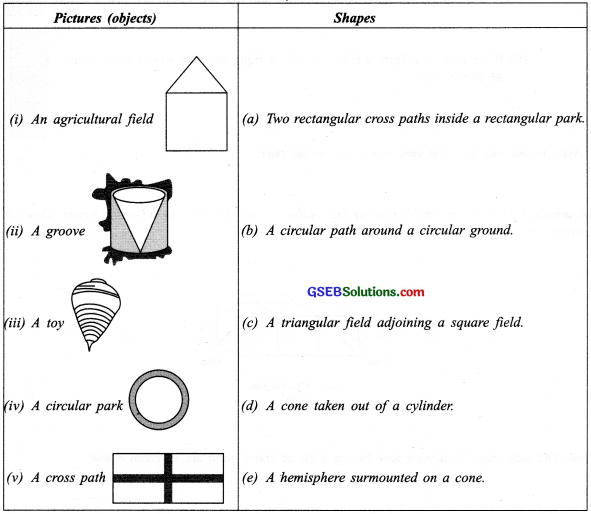# GSEB Solutions Class 8 Maths Chapter 10 Visualizing Solid Shapes InText Questions

Gujarat Board GSEB Textbook Solutions Class 8 Maths Chapter 10 Visualizing Solid Shapes InText Questions and Answers.

## Gujarat Board Textbook Solutions Class 8 Maths Chapter 10 Visualizing Solid Shapes InText Questions

Try These (Page 153)

Question 1.
Match the following: (First one has been done for you.)Solution:
(A) → (ii) → (c)
(B) → (iii) → (g)
(C) → (i) → (b)
(D) → (iv) → (h)
(E) → (v) → (f)
(F) → (vii) → (d)
(G) → (vi) → (e)
(H) → (viii) → (a)Try These (Page 154)

Question 1.
Match the following pictures (objects) with their shapes:Solution:
(i) → (c)
(ii) → (d)
(iii) → (e)
(iv) → (b)
(v) → (a)